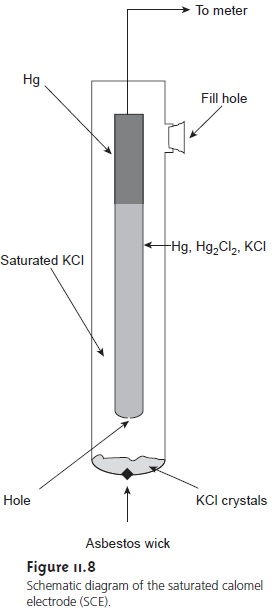Home | | Modern Analytical Chemistry | Reference Electrodes - Potentiometric Methods of Analysis

# Reference Electrodes - Potentiometric Methods of AnalysisPotentiometric electrochemical cells are constructed such that one of the half-cells provides a known reference potential, and the potential of the other half-cell indi- cates the analyte’s concentration.

Reference Electrodes

Potentiometric electrochemical cells are constructed such that one of the half-cells provides a known reference potential, and the potential of the other half-cell indi- cates the analyte’s concentration. By convention, the reference electrode is taken to be the anode; thus, the shorthand notation for a potentiometric electrochemical cell isThe ideal reference electrode must provide a stable potential so that any change in Ecell is attributed to the indicator electrode, and, therefore, to a change in the ana- lyte’s concentration. In addition, the ideal reference electrode should be easy to make and to use. Three common reference electrodes are discussed in this section.

## Standard Hydrogen Electrode

The standard hydrogen electrode (SHE) is rarely used for routine analytical work, but is important because it is the reference elec- trode used to establish standard-state potentials for other half-reactions. The SHE consists of a Pt electrode immersed in a solution in which the hydrogen ion activity is 1.00 and in which H2 gas is bubbled at a pressure of 1 atm (Figure 11.7). A con- ventional salt bridge connects the SHE to the indicator half-cell.The shorthand no- tation for the standard hydrogen electrode is

Pt(s), H2 (g, 1 atm) | H+ (aq, a = 1.00) ||

and the standard-state potential for the reaction

2H+(aq)+ e– < = = = = > H2(g)

is, by definition, 0.00 V for all temperatures. Despite its importance as the funda- mental reference electrode against which all other potentials are measured, the SHE is rarely used because it is difficult to prepare and inconvenient to use.

## Calomel Electrodes

Calomel reference electrodes are based on the redox couple between Hg2Cl2 and Hg (calomel is a common name for Hg2Cl2).

Hg2Cl2(s) +2e– < = = = = > 2Hg(l) + 2Cl(aq)

The Nernst equation for the calomel electrode isThe potential of a calomel electrode, therefore, is determined by the concentration of Cl.

The saturated calomel electrode (SCE), which is constructed using an aqueous solution saturated with KCl, has a potential at 25 °C of +0.2444 V. A typical SCE is shown in Figure 11.8 and consists of an inner tube, packed with a paste of Hg, Hg2Cl2, and saturated KCl, situated within a second tube filled with a saturated so- lution of KCl. A small hole connects the two tubes, and an asbestos fiber serves as a salt bridge to the solution in which the SCE is immersed. The stopper in the outer tube may be removed when additional saturated KCl is needed. The shorthand no- tation for this cell isThe SCE has the advantage that the concentration of Cl, and, therefore, the poten- tial of the electrode, remains constant even if the KCl solution partially evaporates. On the other hand, a significant disadvantage of the SCE is that the solubility of KCl is sensitive to a change in temperature. At higher temperatures the concentration of Cl increases, and the electrode’s potential decreases. For example, the potential of the SCE at 35 °C is +0.2376 V. Electrodes containing unsaturated solutions of KCl have potentials that are less temperature-dependent, but experience a change in po- tential if the concentration of KCl increases due to evaporation. Another disadvan- tage to calomel electrodes is that they cannot be used at temperatures above 80 °C.

## Silver/SilverChlorideElectrodes

Another common reference electrode is the silver/silver chloride electrode, which is based on the redox couple between AgCl and Ag.

AgCl(s)+ e– < = = = = > Ag(s)+ Cl(aq)

As with the saturated calomel electrode, the potential of the Ag/AgCl electrode is determined by the concentration of Cl used in its preparation.When prepared using a saturated solution of KCl, the Ag/AgCl electrode has a potential of +0.197 V at 25 °C. Another common Ag/AgCl electrode uses a so- lution of 3.5 M KCl and has a potential of +0.205 at 25 °C. The Ag/AgCl elec- trode prepared with saturated KCl, of course, is more temperature-sensitive than one prepared with an unsaturated solution of KCl.A typical Ag/AgCl electrode is shown in Figure 11.9 and consists of a sil- ver wire, the end of which is coated with a thin film of AgCl. The wire is im- mersed in a solution that contains the desired concentration of KCl and that is saturated with AgCl. A porous plug serves as the salt bridge. The shorthand notation for the cell is

Ag(s) | AgCl (sat’d), KCl (x M) ||

where x is the concentration of KCl.

In comparison to the SCE the Ag/AgCl electrode has the advantage of being useful at higher temperatures. On the other hand, the Ag/AgCl electrode is more prone to reacting with solutions to form insoluble silver complexes that may plug the salt bridge between the electrode and the solution.

Study Material, Lecturing Notes, Assignment, Reference, Wiki description explanation, brief detail
Modern Analytical Chemistry: Electrochemical Methods of Analysis : Reference Electrodes - Potentiometric Methods of Analysis |